# How to Use Python NumPy Random Function?

When the value of the number changes in each execution of the script, then that number is called a random number. The random numbers are mainly used for the various types of testing and sampling. Many ways exist in Python to generate the random number, and using a random module of the NumPy library is one way to do it. Many functions exist in random module to generate random numbers, such as rand(), randint(), random(), etc. The uses of the random() function of the random module to generate random numbers in Python are shown in this tutorial.

## Generate random numbers using the random() function

The syntax of the random() function of the random module is given below.

## Syntax:

array numpy.random.random(size=None)

This function can take one optional argument, and the default value of this argument is None.  Any integer or the tuple of integers can be given as the argument value that defined the shape of the array that will be returned as the output. If no argument value is provided, then a single floating number will be returned instead of an array. Different uses of the random() function are shown below.

## Example-1: Use of random() function without any argument value

The following example shows the use of random() function without any argument that generates a scalar random number. The returned value of this function is printed later.

# import NumPy library

import numpy as np

# Call random() function without argument

random_number = np.random.random()

# Print the random value

print ("The output of the random() function is: ", random_number)

Output:

The following output will appear after executing the above script. It shows fractional random numbers.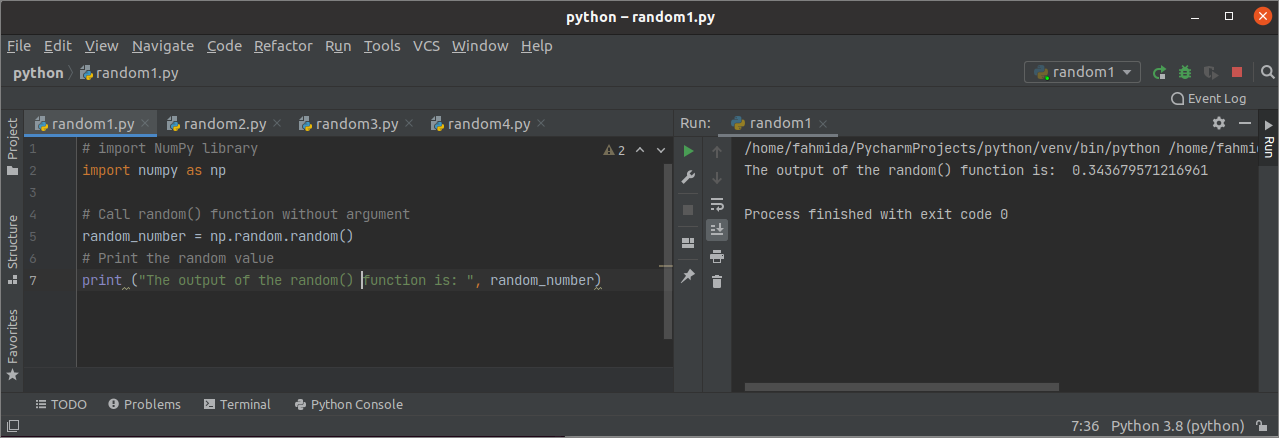## Example-2: Use of random() function with an integer

The following example shows the use of the random() function with an integer in the value of the size argument. Here, 4 is set to the size argument. The means that the random() function will generate an array of four fractional random numbers. The output of the function is printed later.

# import NumPy library

import numpy as np

# Create an array of 4 random numbers

np_array = np.random.random(size=4)

# Print the array

print("The output of the random() function is:
"
, np_array)

Output:

The following output will appear after executing the above script. It shows the one-dimensional array of fractional numbers.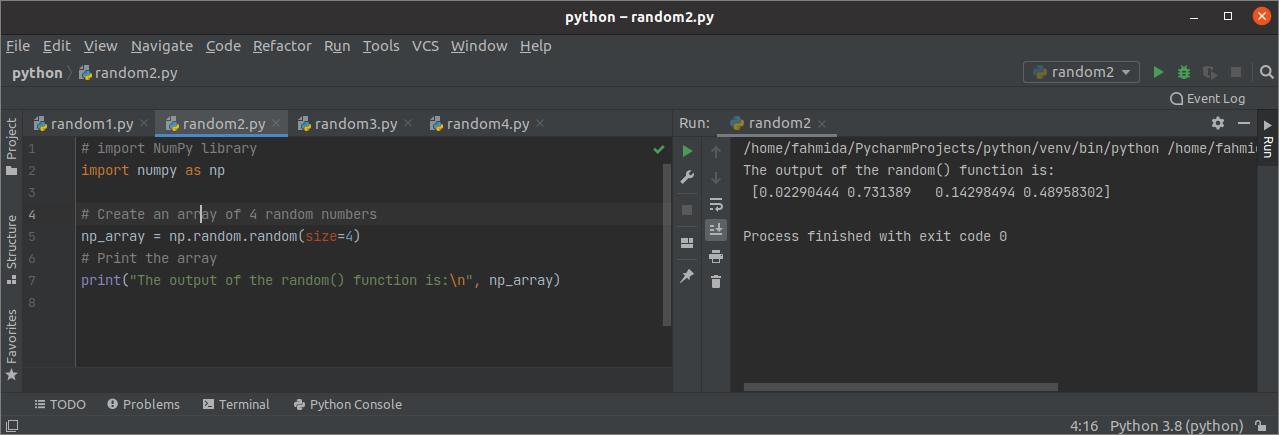## Example-3: Use of random() function with a tuple of two integers

The following example shows how the random() function can be used to create a two-dimensional array of fractional random numbers. Here, (2,5) is used as the value of size argument, and the function will return a two-dimensional array of fractional numbers with 2 rows and 5 columns.

# import NumPy library

import numpy as np

# Create a two-dimensional array of random numbers

np_array = np.random.random(size=(2, 5))

# Print the array

print("The output of the random() function is:
"
, np_array)

Output:

The following output will appear after executing the above script. It shows a two-dimensional array of fractional random numbers.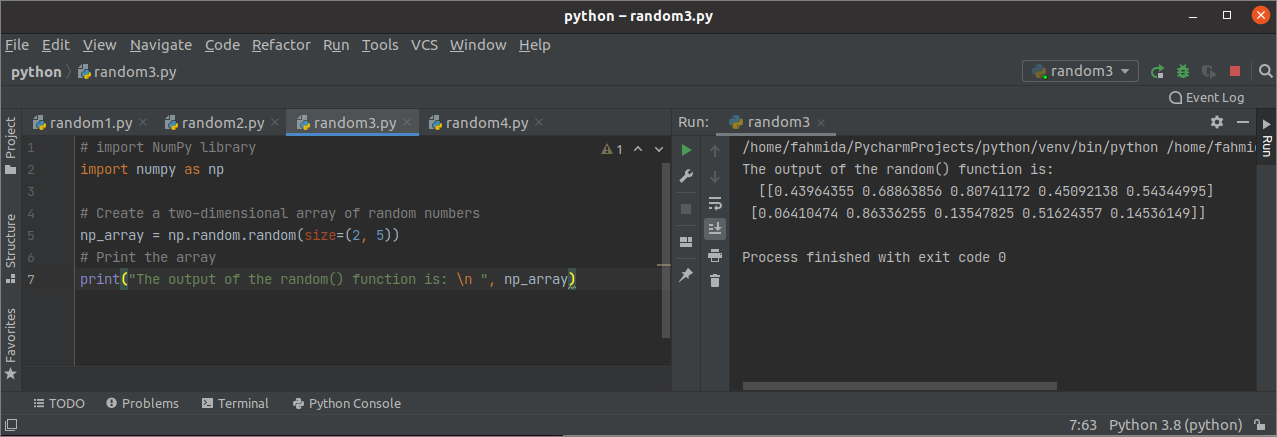## Example-4: Use of random() function with a tuple of three integers

The following example shows how the random() function can be used to create a three-dimensional array of fractional random numbers. Here, (2,3,4) is used as the value of size argument, and the function will return a three-dimensional array of fractional numbers with 3 rows and 4 columns of 2 times.

# import NumPy library

import numpy as np

# Create a three-dimensional array of random numbers

np_array = np.random.random(size=(2, 3, 4))

# Print the array

print("The output of the random() function is:
"
, np_array)

Output:

The following output will appear after executing the above script. It shows a three-dimensional array of fractional random numbers.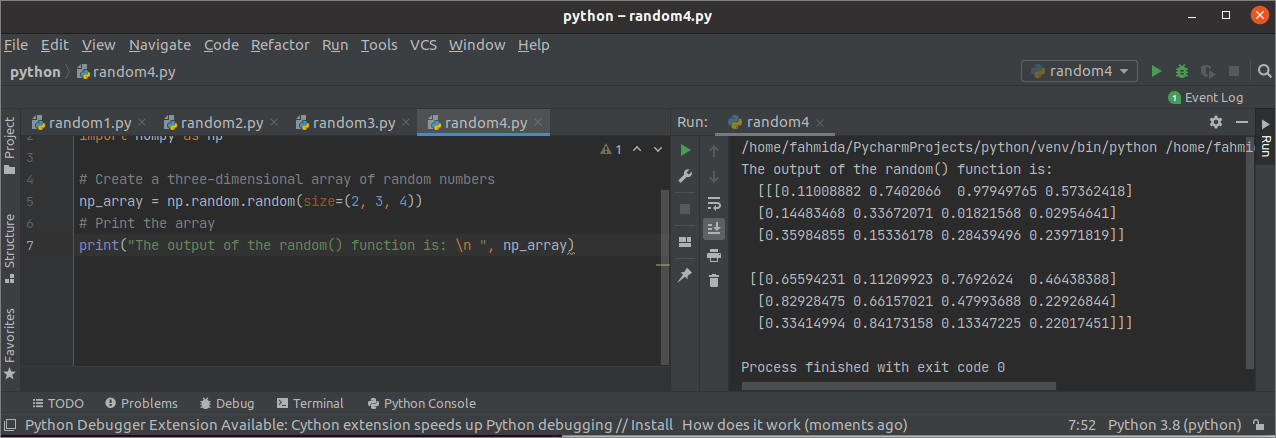## Example-5: Use of random() function to generate coin flips

The following example shows the way to generate coin flips using random numbers. A NumPy array of 10 random fractional numbers has been created using the random() function. heads array has been created with the boolean values by comparing the array values with 0.7.  Next, the values of the heads array and the total number of True values in the heads array have been printed.

# Import NumPy library

import numpy as np

# Create an array of 10 random numbers

np_array = np.random.random(10)

# Create the coin flips array based on array values

print("The values of head array are:
"

# Print the number of heads

print("

Output:

The following similar output will appear after executing the script. The different outputs will be generated at different times for random numbers. According to the following output, the total number of True values is 4.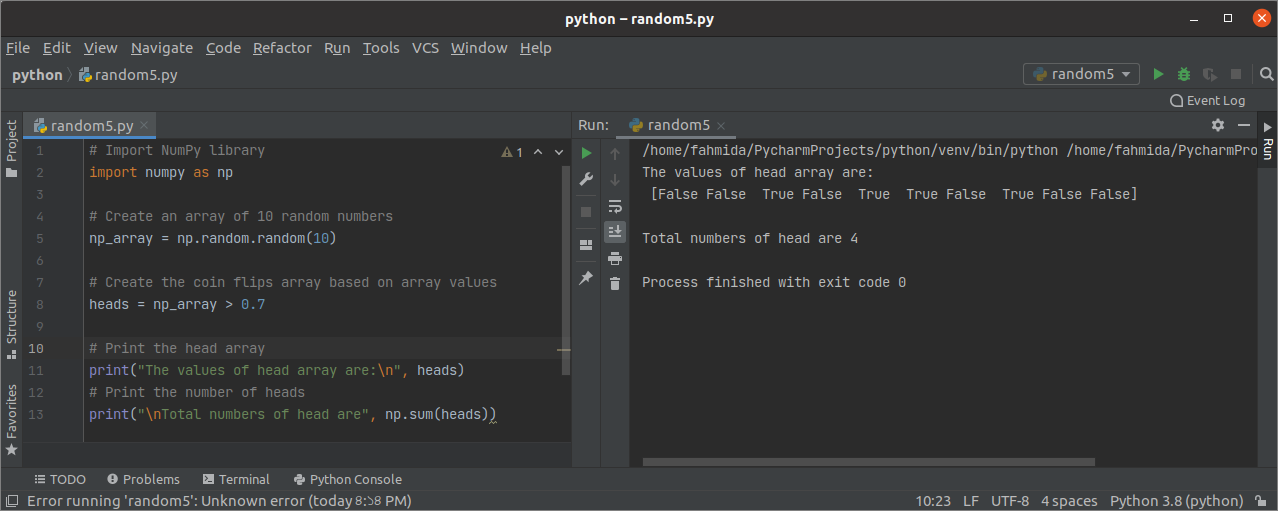## Example-6: Use of random() function for plotting

The following example shows the way to generate the plots of a chart using the random() function. Here, the values of the x-axis have been generated using random() and sort() functions. The values of the y-axis have been generated using arange() function. Next, the plot() function of matplotlib.pyplot has been used to draw the plots of the chart. show() function has been used to display the chart.

# Import necessary libraries

import numpy as np

import matplotlib.pyplot as plt

# Create sorted array of random numbers

x_axis = np.sort(np.random.random(500000))

# Create x-axis for CDF(Continues Probability Distribution)

y_axis = np.arange(1, 500000)

# Plot CDF from random numbers

plt.plot(x_axis[::500], y_axis[::500], marker='.', markersize=5, color='red')

# Display the chart

plt.show()

Output:

The following similar output will appear after executing the above script.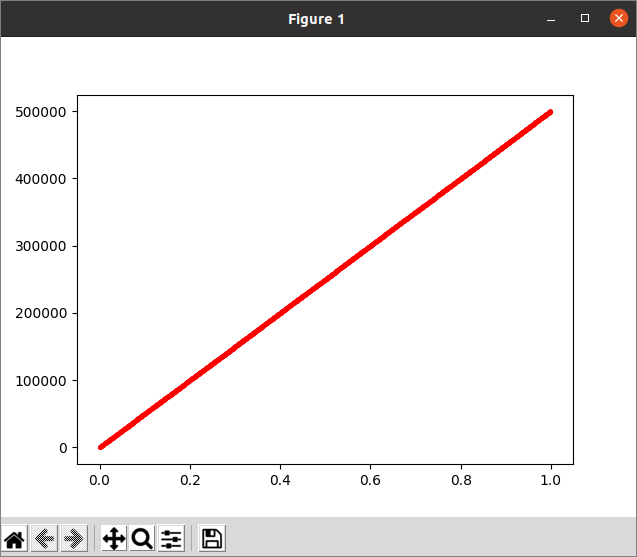## Conclusion

The random() function is a very useful function of Python to perform different types of tasks. Various uses of the random() function have been shown in this tutorial using multiple examples. The purpose of using this function will be cleared for the readers after practicing the examples of this tutorial properly.

sources news post: linuxhint.com